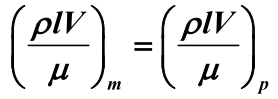cancel
Showing results for
Did you mean:
cancel
Showing results for
Did you mean:

SOLVED

## How to type a formular for demonstrition purpose?

I want to type in a formular in MathCAD just for demonstration, i don't want MathCAD to run or calculate it.

For example following equetions: it throw me error that variable is not defined.1 ACCEPTED SOLUTION

Accepted Solutions

## Re: How to type a formular for demonstrition purpose?4 REPLIES 4

## Re: How to type a formular for demonstrition purpose?

@Mirui wrote:

I want to type in a formular in MathCAD just for demonstration, i don't want MathCAD to run or calculate it.

For example following equetions: it throw me error that variable is not defined.## Re: How to type a formular for demonstrition purpose?

@Mirui wrote:

I want to type in a formular in MathCAD just for demonstration, i don't want MathCAD to run or calculate it.

For example following equetions: it throw me error that variable is not defined.## Re: How to type a formular for demonstrition purpose?Highlighted

Great!!!